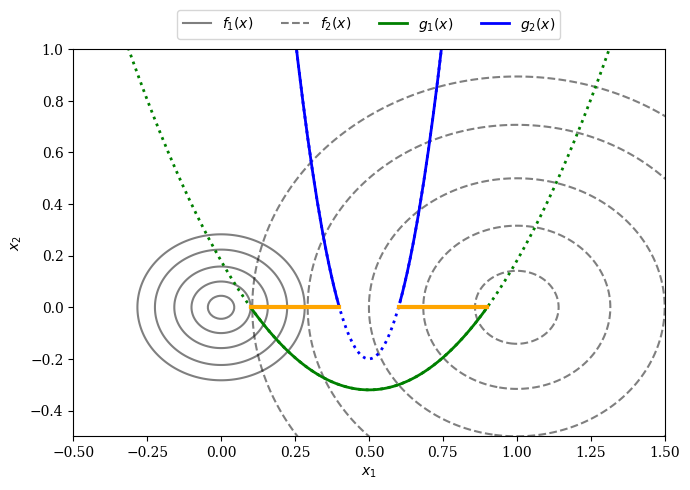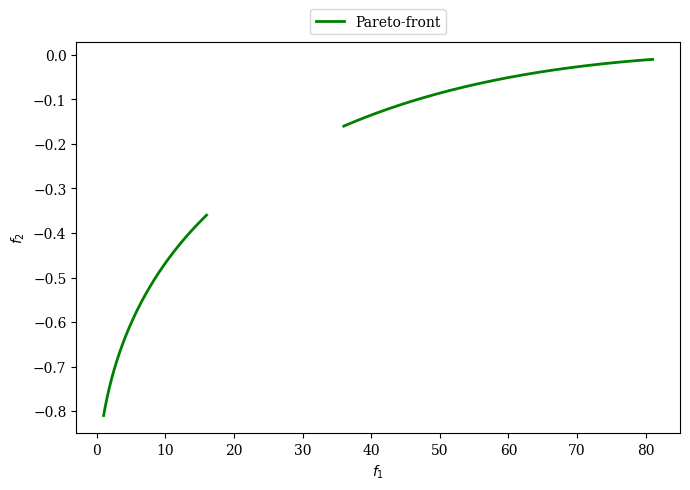Version: 0.5.0

# Part I: A Constrained Bi-objective Optimization Problem¶

In the following, we investigate exemplarily a bi-objective optimization with two constraints. We have tried to select a suitable optimization problem with enough complexity for demonstration purposes, but not too difficult to lose track of the overall idea. Its definition is given by:

As already discussed in the Preface, let an optimization problem be defined by:

\begin{align} \begin{split} \min \quad& f_{m}(x) \quad \quad \quad \quad m = 1,..,M \4pt] \text{s.t.} \quad& g_{j}(x) \leq 0 \quad \; \; \, \quad j = 1,..,J \\[2pt] \quad& h_{k}(x) = 0 \quad \; \; \quad k = 1,..,K \\[4pt] \quad& x_{i}^{L} \leq x_{i} \leq x_{i}^{U} \quad i = 1,..,N \\[2pt] \quad& x \in \Omega \end{split} \end{align} The the example problem to be solved in this getting started guide is given by: \begin{align} \begin{split} \min \;\; & f_1(x) = 100 \,(x_1^2 + x_2^2) \\ \max \;\; & f_2(x) = -(x_1-1)^2 - x_2^2 \\[1mm] \text{s.t.} \;\; & g_1(x) = 2 \, (x_1 - 0.1) \, (x_1 - 0.9) \leq 0\\ & g_2(x) = 20 \, (x_1 - 0.4) \, (x_1 - 0.6) \geq 0\\[1mm] & -2 \leq x_1 \leq 2 \\ & -2 \leq x_2 \leq 2\\[1mm] & x \in \mathbb{R} \end{split} \end{align} The problem consists of two objectives ($$M=2$$) where $$f_1(x)$$ is minimized and $$f_2(x)$$ maximized. The optimization is subject to two inequality constraints ($$J=2$$) where $$g_1(x)$$ is formulated as a less than and $$g_2(x)$$ as a greater than constraint. The problem is defined with respect to two variables ($$N=2$$), $$x_1$$ and $$x_2$$, both in the range $$[-2,2]$$. The problem does not contain any equality constraints ($$K=0$$). Note Next, we derive the optimum for the given optimization problem. It is worth pointing out that this is not a requirement for pymoo and is just done for verification purposes here. Moreover, this is a valuable exercise to understand the design and objective space mapping. Let us analyze where the Pareto-optimal solutions have to lie. The first objective $$f_1$$ is minimized at $$(0,0)$$, whereas the second object $$f_2$$ at $$(1, 0)$$. Because both functions are of quadratic nature, the optimum is given by a straight line between the two optima. This means all Pareto-optimal solutions (ignoring the constraints for now) have in common that $$x_2=0$$ and $$x_1 \in (0,1)$$. The first constraint only relies on $$x_1$$ and is satisfied if $$x_1 \in (0.1,0.9)$$. The second constraint $$g_2$$ is satisfied for $$x_1 \in (0.4,0.6)$$. This means analytically, the pareto-optimal set is given by $$PS = \{(x_1, x_2) \,|\, (0.1 \leq x_1 \leq 0.4) \lor (0.6 \leq x_1 \leq 0.9) \, \land \, x_2 = 0\}$$. The figure below shows the problem’s functions in the design space and might help to see the relationship between the functions intuitively. The contour lines of the objective function $$f_1(x)$$ is represented by a solid and $$f_2(x)$$ by a dashed line. The constraints $$g_1(x)$$ and $$g_2(x)$$ are parabolas which intersect the $$x_1$$-axis at $$(0.1, 0.9)$$ and $$(0.4, 0.6)$$. A thick orange line illustrates the Pareto-optimal set. When considering both constraints together, the Pareto-set shown in orange is split into two parts as analytically derived above. :  import numpy as np X1, X2 = np.meshgrid(np.linspace(-2, 2, 500), np.linspace(-2, 2, 500)) F1 = X1**2 + X2**2 F2 = (X1-1)**2 + X2**2 G = X1**2 - X1 + 3/16 G1 = 2 * (X1 - 0.1) * (X1 - 0.9) G2 = 20 * (X1 - 0.4) * (X1 - 0.6) import matplotlib.pyplot as plt plt.rc('font', family='serif') levels = [0.02, 0.1, 0.25, 0.5, 0.8] plt.figure(figsize=(7, 5)) CS = plt.contour(X1, X2, F1, levels, colors='black', alpha=0.5) CS.collections.set_label("f_1(x)") CS = plt.contour(X1, X2, F2, levels, linestyles="dashed", colors='black', alpha=0.5) CS.collections.set_label("f_2(x)") plt.plot(X1, G1, linewidth=2.0, color="green", linestyle='dotted') plt.plot(X1[G1<0], G1[G1<0], label="g_1(x)", linewidth=2.0, color="green") plt.plot(X1, G2, linewidth=2.0, color="blue", linestyle='dotted') plt.plot(X1[X1>0.6], G2[X1>0.6], label="g_2(x)",linewidth=2.0, color="blue") plt.plot(X1[X1<0.4], G2[X1<0.4], linewidth=2.0, color="blue") plt.plot(np.linspace(0.1,0.4,100), np.zeros(100),linewidth=3.0, color="orange") plt.plot(np.linspace(0.6,0.9,100), np.zeros(100),linewidth=3.0, color="orange") plt.xlim(-0.5, 1.5) plt.ylim(-0.5, 1) plt.xlabel("x_1") plt.ylabel("x_2") plt.legend(loc='upper center', bbox_to_anchor=(0.5, 1.12), ncol=4, fancybox=True, shadow=False) plt.tight_layout() plt.show()Next, we derive the Pareto-front by mapping the Pareto-set to the objective space. The Pareto-front equation is based on $$f_2$$ depending on the variable of $$f_1$$. We know that at the optimum $$x_2=0$$ which means we can simplify the objective functions to $$f_1(x) = 100 \; x_1^2$$ and $$f_2(x) = -(x_1-1)^2$$. The first objective $$f_1$$ can be reformulated to $$x_1 = \sqrt{\frac{f_1}{100}}$$ and then be put into the second objective which results in \[f_2 = -\left(\sqrt{\frac{f_1}{100}}-1\right)^2

The equation defines the shape, however, next all possible values for $$f_1$$ need to be defined. As shown before the Pareto-set is defined for $$(0.1 \leq x_1 \leq 0.4) \lor (0.6 \leq x_1 \leq 0.9) \, \land \, x_2 = 0$$. If we plug in the values for $$x_1$$ into $$f_1$$ we get the points of interest $$[1,16]$$ and $$[36,81]$$. Thus the Pareto-front is given by:

:

import numpy as np
import matplotlib.pyplot as plt

plt.figure(figsize=(7, 5))

f2 = lambda f1: - ((f1/100) ** 0.5 - 1)**2
F1_a, F1_b = np.linspace(1, 16, 300), np.linspace(36, 81, 300)
F2_a, F2_b = f2(F1_a), f2(F1_b)

plt.rc('font', family='serif')
plt.plot(F1_a,F2_a, linewidth=2.0, color="green", label="Pareto-front")
plt.plot(F1_b,F2_b, linewidth=2.0, color="green")

plt.xlabel("$f_1$")
plt.ylabel("$f_2$")

plt.legend(loc='upper center', bbox_to_anchor=(0.5, 1.10),